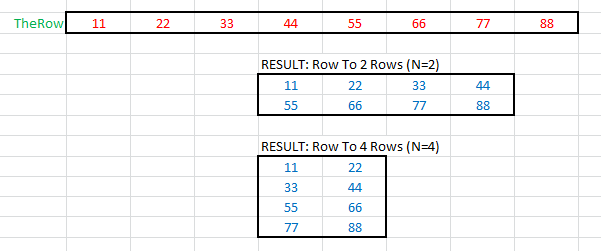Transforming Row And Column Ranges To A Matrix

This page describes formulas for converting a row or column to a matrix.Introduction

You may have data in a row or column that you want to transform into a matrix. This page describes formulas you can use to accomplish that. First, we'll look at transforming a column to a matrix. Next, we'll look at transforming a row to a matrix.Column To Matrix

Suppose you have a single column of data in a range named TheCol2. This is a single column spanning several rows. To convert it to a 2xN matrix, two rows and N columns where N = (rows of TheCol2 divided by 2), you can use the formula below. This formula is entered cell D9. Change the reference to \$D\$9 to the first cell in which you enter this formula.

=OFFSET(TheCol2,COLUMN()-COLUMN(\$D\$9)+((ROW()-ROW(\$D\$9))*(ROWS(TheCol2)/2)),0,1,1)

Copy this formula across the row into N cells where N is one half the number of columns of TheCol2. Then, copy these cells down one row. For example, if TheCol2 is B8:B16 and you enter the formula initially in cell D9, you would copy the formula across to G9 and then copy D9:G9 down one row to fill D9:G10. If you want more rows and fewer columns, change ROWS(TheCol2)/2 to ROWS(TheCol2)/N where N is the number of rows to fill. A screen shot of the data TheCol2 and the result matrices are shown below:Row To Matrix

A similar operation can be performed when the source data is in a single row. Suppose you have a single row of data in the range B22:I22, with a defined name of TheRow. To convert this to a matrix, enter the following formula in cell E25:

=OFFSET(TheRow,0,COLUMN()-COLUMN(\$E\$25)+(ROW()-ROW(\$E\$25))*(COLUMNS(TheRow)/2),1,1)

Change the reference in the formula to \$E\$25 to the first cell in which you enter this formula. Copy this formula across to H25, or half the number of cells in the range TheRow. Then, copy those cells down one row. The formula should now be entered into the range E25:H26. To span more rows and fewer columns, change COLUMNS(TheRow)/2 to COLUMNS(TheRow)/N where N is the number of rows in the resulting matrix. A screen shot of the data and resulting matrices is shown below:For formulas that do the reverse of these functions, namely create a vector (row or column) from a matrix, see the Matrix To Vector page.You can download the file with all the example formulas on this page.This page last updated: 31-January-2011.

-->